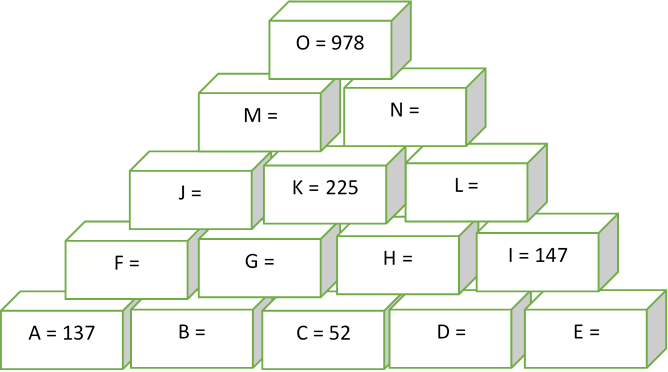# Reverse Pascal Triangle

Algebra Level 2Every brick in this pyramid contains a number that is the sum of the two numbers in the two bricks below it. What is the value of $B$?

×